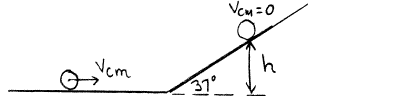Problem: A thin-walled hollow sphere with mass M = 2.00 kg and radius R = 0.05 00 m has a moment of inertia of I = 2/3 MR2 for rotation about an axis through its center. Initially the sphere is rolling without slipping on a level horizontal surface and its center of mass has a translational speed of vcm = 8.00 m/s. The sphere then rolls without slipping up a ramp that is inclined at 37° above the horizontal. What is the magnitude of the friction force that the ramp exerts on the sphere while the sphere is rolling up the ramp?

FREE Expert Solution
82% (177 ratings)
Problem Details

A thin-walled hollow sphere with mass M = 2.00 kg and radius R = 0.05 00 m has a moment of inertia of I = 2/3 MR2 for rotation about an axis through its center. Initially the sphere is rolling without slipping on a level horizontal surface and its center of mass has a translational speed of vcm = 8.00 m/s. The sphere then rolls without slipping up a ramp that is inclined at 37° above the horizontal. What is the magnitude of the friction force that the ramp exerts on the sphere while the sphere is rolling up the ramp?